Next:Properties of Information MeasuresUp:Information and Divergence Measures

Relative Information and Inaccuracy

Kullback and Leibler's (1951)  measure of information associated with the probability distributions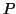and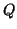is given by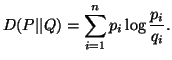(2.1)

The measure (2.1) has many names given by different authors such as, relative information, directed divergence, cross entropy, function of discrimination etc.. Here we shall refer it "relative information". It has found many applications in setting important theorems in information theory and statistics.

The Kerridge's (1961)  measure of information generally referred as inaccuracy associated with two probability distributions is given by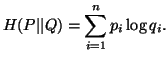(2.2)

Various authors studied characterizations and properties of the measures (2.1) and (2.2) separately. Here we present their joint study.

Let us consider a measure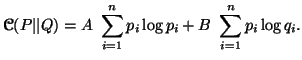(2.3)

Then for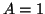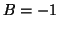, we get (2.1) and for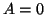, we get (2.2).

For simplicity, let define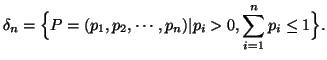The following theorem give axiomatic characterization of the measure (2.3)

Theorem 2.1. Let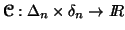(reals) be a function satisfying the following axioms:

A1. (Symmetry).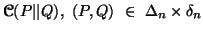is symmetric for every permutation of elements in Q.
A2. (Branching). We have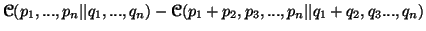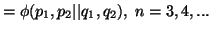where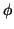is a continuous function defined over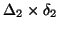.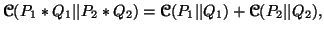where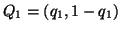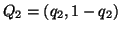,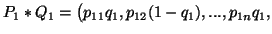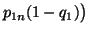,
etc..

Then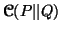is given by (2.3).

By considering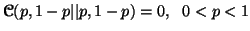and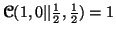in (2.3) we get (2.1). Again taking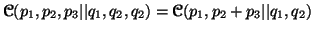and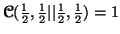we get (2.2).

Measure (2.3) can also be characterized by different approaches using functional equation or axiomatic aprroach. In functional's equation approach, the following two equations are frequently used: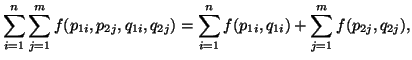and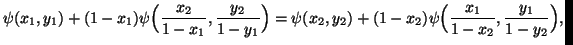for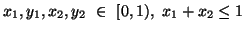and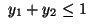, where the fuctions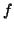and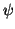are considered under certain regularity conditions.

For more details refer to Mathai and Rathai (1975) , Autar (1975) , Taneja (1979)  etc..

Subsections

21-06-2001
Inder Jeet Taneja
Departamento de Matemática - UFSC
88.040-900 Florianópolis, SC - Brazil